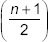# User ForumSubject :IMO    Class : Class 8

State ‘T’ for true and ‘F’ for false.
(i) The square root of 0.9 is 0.3.
(ii) The square root of a perfect square of n digits will havedigits, if n is odd.
(iii) All numbers of a pythagorean triplet are odd.
(iv) There are 200 natural numbers between 1002 and 1012.

 (i) (ii) (iii) (iv) A T F T F B F T F T C T T F F D F T T F

## Ans 1:

Class : Class 8
2) F,T,F,T is the right answer

Class : Class 8